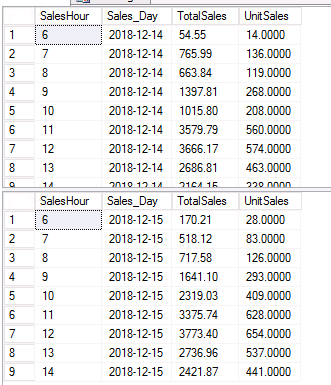# Join 2 result sets, grouped by date and by hour

Hi,

We are comparing sales by hour for our store, and I am currently using 2 separate queries.

I'd like to combine these results into one result set, by making the 2 queries derived tables, and joining them.   So the second result set in the attached screenshot would be additional columns added to the first result set.   I was having a hard time getting this to work correctly.

Thanks in advance for a solution on this.``````USE STORESQL

SELECT

--I.F22,
--R.F1041 AS ITEM_DESCR,
--R.F01 AS ITEM_NO,
DATEPART(HOUR, R.F253) as SalesHour,
CONVERT(DATE,R.F253) AS Sales_Day,
SUM(R.F65) AS TotalSales,
SUM(R.F64) AS UnitSales
FROM

SAL_REG_SAV R
JOIN SAL_HDR_SAV H ON
R.F1505 = H.F1505
AND R.F1056 = H.F1056
AND R.F1057 = H.F1057
AND R.F1032 = H.F1032
JOIN POS_TAB P ON R.F01 = P.F01
JOIN OBJ_TAB I ON R.F01 = I.F01

WHERE
R.F1505 = '12-14-2018'
AND R.F1056 = '047'

GROUP BY  DATEPART(HOUR, R.F253), CONVERT(DATE,R.F253)
ORDER BY CONVERT(DATE,R.F253), DATEPART(HOUR, R.F253)

SELECT

--I.F22,
--R.F1041 AS ITEM_DESCR,
--R.F01 AS ITEM_NO,
DATEPART(HOUR, R.F253) as SalesHour,
CONVERT(DATE,R.F253) AS Sales_Day,
SUM(R.F65) AS TotalSales,
SUM(R.F64) AS UnitSales
FROM

SAL_REG_SAV R
JOIN SAL_HDR_SAV H ON
R.F1505 = H.F1505
AND R.F1056 = H.F1056
AND R.F1057 = H.F1057
AND R.F1032 = H.F1032
JOIN POS_TAB P ON R.F01 = P.F01
JOIN OBJ_TAB I ON R.F01 = I.F01

WHERE
R.F1505 = '12-15-2018'
AND R.F1056 = '047'

GROUP BY  DATEPART(HOUR, R.F253), CONVERT(DATE,R.F253)
ORDER BY CONVERT(DATE,R.F253), DATEPART(HOUR, R.F253)
``````
Microsoft SQL ServerLast CommentSharath STHIS SOLUTION IS ONLY AVAILABLE TO MEMBERS.
View this solution by signing up for a free trial.
Members can start a 7-Day free trial and enjoy unlimited access to the platform.Thanks that worked.Microsoft SQL Server

Microsoft SQL Server is a suite of relational database management system (RDBMS) products providing multi-user database access functionality.SQL Server is available in multiple versions, typically identified by release year, and versions are subdivided into editions to distinguish between product functionality. Component services include integration (SSIS), reporting (SSRS), analysis (SSAS), data quality, master data, T-SQL and performance tuning.

171K
Questions
--
Followers
--
Top Experts
Get a personalized solution from industry experts

TRUSTED BY